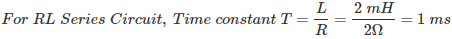# MCQs on Electric Circuits

##### Page 9 of 63. Go to page 1 2 3 4 5 6 7 8 9 10 11 12 13 14 15 16 17 18 19 20 21 22 23 24 25 26 27 28 29 30 31 32 33 34 35 36 37 38 39 40 41 42 43 44 45 46 47 48 49 50 51 52 53 54 55 56 57 58 59 60 61 62 63
01․ In RC series circuit R = 2Ω, C = 2μF and 10V dc is applied. Then what is the value of current?
0A
2A
5A
10A

For DC voltage capacitor acts as an open circuit or have infinite impedance at steady state condition. So, it will not allow any current through it.

02․ Time constant of RL series circuit is
2L/R
RC
L/R
2RC

Time constant of RL series circuit is L/R.

03․ Time constant of RC series circuit is
L/R
2RC
2L/R
RC

Time constant of RC series circuit is RC.

04․ In RL series circuit R=2Ω and L=2mH. What is the value of time constant?
1msec
2msec
4msec
100sec05․ Time constant is the time taken for response to rise -------of maximum value?
100%
90%
63.2%
68.3%

Time constant can be defined either with respect to charging or discharging action of energy storage element. Time constant is the time taken for response to rise 63.2% of maximum value or decrease 37.8% of its maximum value.

06․ Which of the following is the different one in terms of measurements?
L/R
RC
2L/R
Q

L/R, RC and 2L/R represents the time constants of different circuit element condition, but Q represents the quality factor of a coil.

07․ In which of the following oscillations are not present?
Under damping
Over damping
Critical damping
Both 2 and 3

In both over and critical damping no oscillations are present. These will directly moves to final value with out making any oscillations quickly. It will undergo true oscillations, approaching zero amplitude due to damping.

08․ In which of the following networks it is not possible to find the transient free response?
RC
RL
RL, RC, and RLC
RLC

In RL, RC, RLC network it is not possible to obtain a transient free response. Since the circuit is having energy storage elements, either charging and discharging actions are present when connected to AC or DC supply.

09․ To find the initial value of a function, the highest power of S in denominator should be ---------- the highest power of S in the numerator.
greater than
less than
equals to
both a and c

If the highest power of S in denominator is greater than the highest power of S in the numerator, then only we get a finite result. If the highest power of S in denominator is less than or equal to the highest power of S in the numerator, then we get infinity as the result by applying initial value theorem.

10․ Final value theorem is not applicable for which of the following?
stable systems
unstable systems
marginally stable systems
Both 2 and 3

To apply final value theorem system should be stable. It is not applicable to unstable of marginally stable system.

<<<7891011>>>